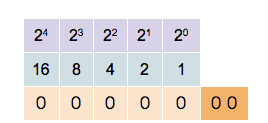## Tag: integer

### Unsigned Integers

Computers use binary representations to store various types of data. In the context of computational physics, it is important to understand how numerical values are stored. To start, let’s take a look at non-negative integer numbers. These unsigned integers can simply be translated into their binary representation. The binary number-format is similar to the all-familiar decimal format with the main difference that there are only two values for the digits, not ten. The two values are 0 and 1. Numbers are written in the same way as decimal numbers only that the place values of each digit are now powers of 2. For example, the following 4-digit numbers show the values of the first four0 0 0 1   decimal value 20 = 1
0 0 1 0   decimal value 21 = 2
0 1 0 0   decimal value 22 = 4
1 0 0 0   decimal value 23 = 8

The binary digits are called bits and in modern computers, the bits are grouped in units of 8. Each unit of 8 bits is called a byte and can contain values between 0 and 28 − 1 = 255. Of course, 255 is not a very large number and for most applications, larger numbers are needed. Most modern computer architectures support integers with 32 bits and 64 bits. Unsigned 32-bit integers range from 0 to 232 − 1 = 4, 294, 967, 295 ≈ 4.3 × 109 and unsigned 64-bit integers range from 0 to 264 − 1 = 18, 446, 744, 073, 709, 551, 615 ≈ 1.8 × 1019. It is worthwhile noting that many GPU architectures currently don’t natively support 64-bit numbers.

The computer’s processor contains registers that can store binary numbers. Thus a 64-bit processor contains 64-bit registers and has machine instructions that perform numerical operations on those registers. As an example, consider the addition operation. In binary, two numbers are added in much the same way as using long addition in decimal. Consider the addition of two 64 bit integers 7013356221863432502 + 884350303838366524. In binary, this is written as follows.

  01100001,01010100,01110010,01010011,01001111,01110010,00010001,00110110
+ 00001100,01000101,11010111,11101010,01110101,01001011,01101011,00111100
---------------------------------------------------------------------------
01101101,10011010,01001010,00111101,11000100,10111101,01111100,01110010


The process of adding two numbers is simple. From right to left, the digits of the two numbers are added. If the result is two or more, there will be a carry-over which is added to the next digit on the left.

You could add integers of any size using this prescription but, of course, in the computer numbers are limited by the number of bits they contain. Consider the following binary addition of (264 − 1) and 1 .

  11111111,11111111,11111111,11111111,11111111,11111111,11111111,11111111
+ 00000000,00000000,00000000,00000000,00000000,00000000,00000000,00000001
---------------------------------------------------------------------------
00000000,00000000,00000000,00000000,00000000,00000000,00000000,00000000


If you were dealing with mathematical integers, you would expect to see an extra digit 1 on the left. The computer cannot store that bit in the register containing the result but stores the extra bit in a special carry flag. In many computer languages, this unintentional overflow will go undetected and the programmer has to take care that numerical operations do not lead to unintended results.

### Signed Integers

The example above shows that adding two non-zero numbers can result in 0. This can be exploited to define negative numbers. In general, given a number a, the negative  − a is defined as the number that solves the equation
a + ( − a) = 0.
Mathematically, the N-bit integers can be seen as the group of integers modulo 2N. This means that for any number a ∈ {0, …, 2N − 1} the number  − a can be defined as
− a = 2N − a ∈ {0, …, 2N − 1}.
By convention, all numbers whose highest value binary bit is zero are considered positive. Those numbers whose highest value bit is one are considered negative. This makes the addition and subtraction of signed integers straightforward as the processor does not need to implement different algorithms for positive or negative numbers. Signed 32-bit integers range from  − 2, 147, 483, 648 to 2, 147, 483, 647, and 64-bit integers range from  − 9, 223, 372, 036, 854, 775, 808 to 9, 223, 372, 036, 854, 775, 807.

This format of storing negative numbers is called the two’s complement format. The reason for this name becomes obvious when observing how to transform a positive number to its negative.

01100001,01010100,01110010,01010011,01001111,01110010,00010001,00110110 (7013356221863432502)
10011110,10101011,10001101,10101100,10110000,10001101,11101110,11001010 (-7013356221863432502)


To invert a number, first, invert all its bits and then add 1. This simple rule of taking the two’s complement can be easily implemented in the processor’s hardware. Because of the simplicity of this prescription, and the fact that adding a negative number follows the same algorithm as adding a positive number, two’s complement is de-facto the only format used to store negative integers on modern processors.

### Exercises

1. Show that taking the two’s complement of an N-bit number a does indeed result in the negative  − a if the addition of two numbers is defined as the addition modulo 2N.
2. Find out how integers are represented in the programming language of your choice. Does this directly reflect the representation of the underlying architecture? I will be writing another post about this topic soon.
3. Most processors have native commands for multiplying two integers. The result of multiplying the numbers in two N-bit registers are stored in two N-bit result registers representing the high and low bits of the result. Show that the resulting 2N bits will always be enough to store the result.
4. Show how the multiplication of two numbers can be implemented using only the bit-shift operator and conditional addition based on the bit that has been shifted out of the register. The bit-shift operator simply shifts all bits of a register to the left or right.